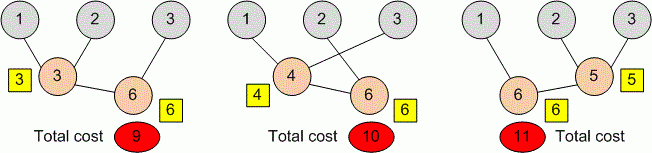Problems

The cost of adding two numbers equals to their sum. For example to add 1 and 10 costs 11. The cost of addition 1 and 2 is 3. We can add numbers in several ways:

• 1 + 2 = 3 (cost = 3), 3 + 3 = 6 (cost = 6), Total = 9
• 1 + 3 = 4 (cost = 4), 2 + 4 = 6 (cost = 6), Total = 10
• 2 + 3 = 5 (cost = 5), 1 + 5 = 6 (cost = 6), Total = 11

We hope you understood the task. You must add all numbers so that the total cost of summation will be the smallest.#### Input

First line contains positive integer n (2n105). Second line contains n nonnegative integers, each no more than 105.

#### Output

Print the minimum total cost of summation.

Time limit 1 second
Memory limit 128 MiB
Input example #1
3
1 2 3

Output example #1
9